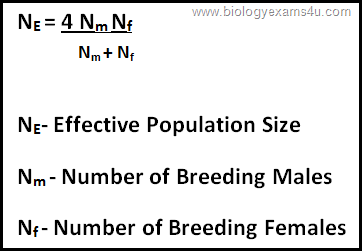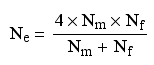Effective Population Size Calculation

If a population consists of breeding males and breeding females, the effective population size is given byWhere Nm is the actual number of breeding males and Nf is the actual number of breeding females.
Practice Problem : 1
In a population of 500 with a 50:50 sex ratio and all individuals breeding, however 250  females bred with 10 males. Then what will be the effective population size?

Applying the equationNm (Number of Breeding Males) = 10
Nf (Number of Breeding Females) = 250
NE- Effective Population Size = (4 x 10 x 250) / (10 +250)
= 10000 / 260
NE = 38.5

Exam Questions:
Effective population size for completely monogamous species having 40 males and 10 females would be (CSIR UGC NET JRF, Dec 2006)
(a) 42 (b) 32 (c) 20 (d) 10
Effective population size (NE) = 4NmNf/ (Nm+Nf)
Nm- Number of breeding males
Nf -Number of breeding females
Here
Nm-40
Nf -10
(NE) = 4NmNf/ (Nm+Nf)
Effective population size (NE) = (4 x 40 x10) / (40 +10) = 1600/50 =  32

We love to hear from you! Leave us a comment.

1.nice for csir preparing exam

2.Thank you so much..

3.4.5.extremely thankful for all your help

6.Very extremely thankful for all your help

7.